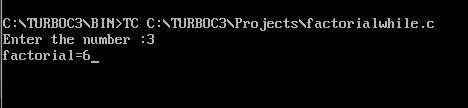UncleCoder.com

UncleCoder.com

Free programming examples and instructions

# C Program to find factorial of a Number using While Loop

## C Program for how to find factorial of a Number using while loop

by Krishna

Posted on 26 Jun 2019 Category: C Views: 5957

C Program to find factorial of a Number Using 'While' Loop

Here we will learn to calculate the factorial of a number using while loop . The factorial of a positive integer n is equal to 1*2*3*...n. This program takes a positive integer from the user and computes factorial using while loop.

PROGRAM

``````#include<stdio.h>
#include<conio.h>
void main()
{
int n,i,f;
f=i=1;

printf("Enter the number: ");
scanf("%d",&n);
while(i<=n)
{
f*=i;
i++;
}
printf("factorial= %d",n,f);
getch();
}``````

OUTPUTRelated Artiles

C program to find sum of first 10 integers using for loop

C program to find factorial of a number using function

C program to generate Star sequence

C program to study while loop

C program to study do-while loop

C Program to Generate Multiplication Table

C program to print Fibonacci series up to 100

C Program to find sum of digits